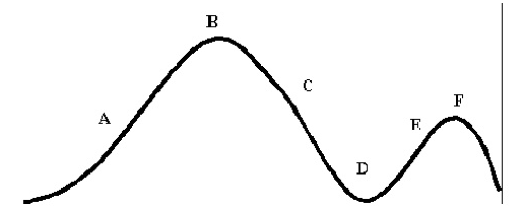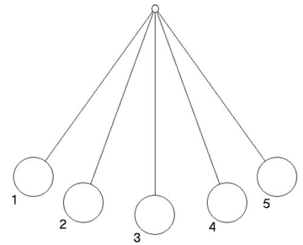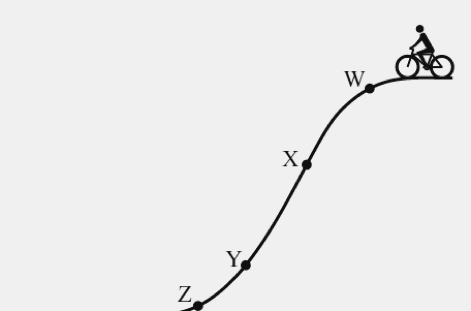# Potential Kinetic Energy Worksheet

Determine whether the objects in the following problems have kinetic or potential energy.

Then choose the correct formula to use:

KE = 1/2 mv^2 (or) PE = mgh = Fwh

### Potential Kinetic Energy Worksheet

1. You serve a volleyball with a mass of 2.1 kg. The ball leaves your hand with a speed of 30 m/s. The ball has ____ energy. Calculate it.

2. A baby carriage is sitting at the top of a hill that is 21 m high. The carriage with the baby weighs 12 N. The carriage has ____ energy. Calculate it.

3. A car is travelling with a velocity of 40 m/s and has a mass of 1120 kg. The car has _______energy. Calculate it.

4. A cinder block is sitting on a platform 20 m high. It weighs 79 N. The block has _____ energy. Calculate it.

5. There is a bell at the top of a tower that is 45 m high. The bell weighs 190 N. The bell has ____ energy. Calculate it.

7. A roller coaster is at the top of a 72 m hill and weighs 966 N. The coaster (at this moment) has ____ energy. Calculate it.

8. What is the kinetic energy of a 3-kilogram ball that is rolling at 2 meters per second?

9. The potential energy of an apple is 6.00 joules. The apple is 3.00-meters high. What is the mass of the apple?

10. What is the kinetic energy of a 1-kilogram ball is thrown into the air with an initial velocity of 30 m/sec?

11. Two objects were lifted by a machine. One object had a mass of 2 kilograms, and was lifted at a speed of2 m/sec. The other had a mass of 4 kilograms and was lifted at a rate of 3 m/sec.

• (a). Which object had more kinetic energy while it was being lifted?
• (b). Which object had more potential energy when it was lifted to a distance of 10 meters? Show your calculation.

12. You are on roller blades on top of a small hill. Your potential energy is equal to 1,000.0 joules. The last time you checked your mass was 60.0 kilograms.

• (a). What is your weight in Newton’s?
• (b). What is the height of the hill?
• (c). If you start skating down this hill, your potential energy will be converted to kinetic energy. At the bottom of the hill, your kinetic energy will be equal to your potential energy at the top. What will be your speed at the bottom of the hill?

13. In a lab investigation, one group of students (group A) measures the speed of a 0.1-kilogram car at 2.5 m/sec at the bottom of a hill. Another group of students (group B) measures the speed of the car at 3 m/sec at the bottom of the hill. The car’s starting position at the top of the hill is one-meter high.

(a). What is the potential energy of the car at the beginning of the experiment before its speed is measured?

(b). Calculate the kinetic energy of the car for group A using the speed (2.5 m/sec) and mass values above.

(c). Calculate the kinetic energy of the car for group B using the speed (3.0 m/sec) and mass values above.

(d). At the bottom of a hill, the kinetic energy of the cars should be equal to the potential energy of the car atthe top of the hill. Are the kinetic energy values for groups A and B equal to, less than, or greater tha h-e potential energy value?

### Potential Kinetic Energy Worksheet 2

1. Imagine the cars were travelling in the roller coaster as shown below. Answer the questions given under the image.• Which letter in the image possesses maximum potential energy? • Which letter represented has least kinetic energy? In which position of the diagram, the cars move with the greatest speed?

2. When the pendulum swings back and forth, it switches between potential and kinetic energy.• At which position the potential energy is maximum? • At which position the kinetic energy is maximum?

3. The diagram represents a bicyclist at the top of a hill with four points labelled W, X, Y, and Z. If the bicyclist does not apply breaks while riding down from the hill. At which point kinetic energy is closest to zero?